# AP Calculus BC Question 43: Answer and Explanation

### Test Information

Question: 43

6. Two particles leave the origin at the same time and move along the y-axis with their respective positions determined by the functions y1 = cos 2t and y2 = 4sin t for 0 < t < 6. For how many values of t do the particles have the same acceleration?

• A. 0
• B. 1
• C. 3
• D. 4

Explanation:

C If you want to find acceleration, all you have to do is take the second derivative of the position functions.

Step 1: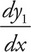= -2sin 2t and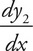= 4cos t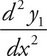= -4cos 2t and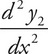= -4sin t

Step 2: Now all we have to do is to graph both of these equations on the same set of axes on a calculator. You should make the window from x = 0 to x = 7 (leave yourself a little room so that you can see the whole range that you need). You should get a picture that looks like the following: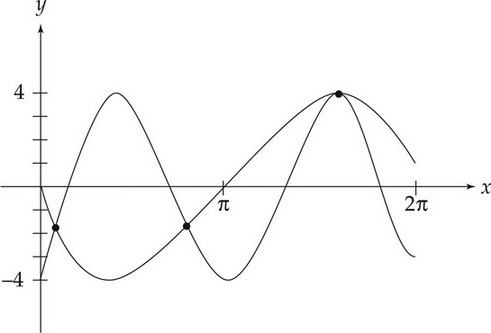Where the graphs intersect, the acceleration is the same. There are three points of intersection.